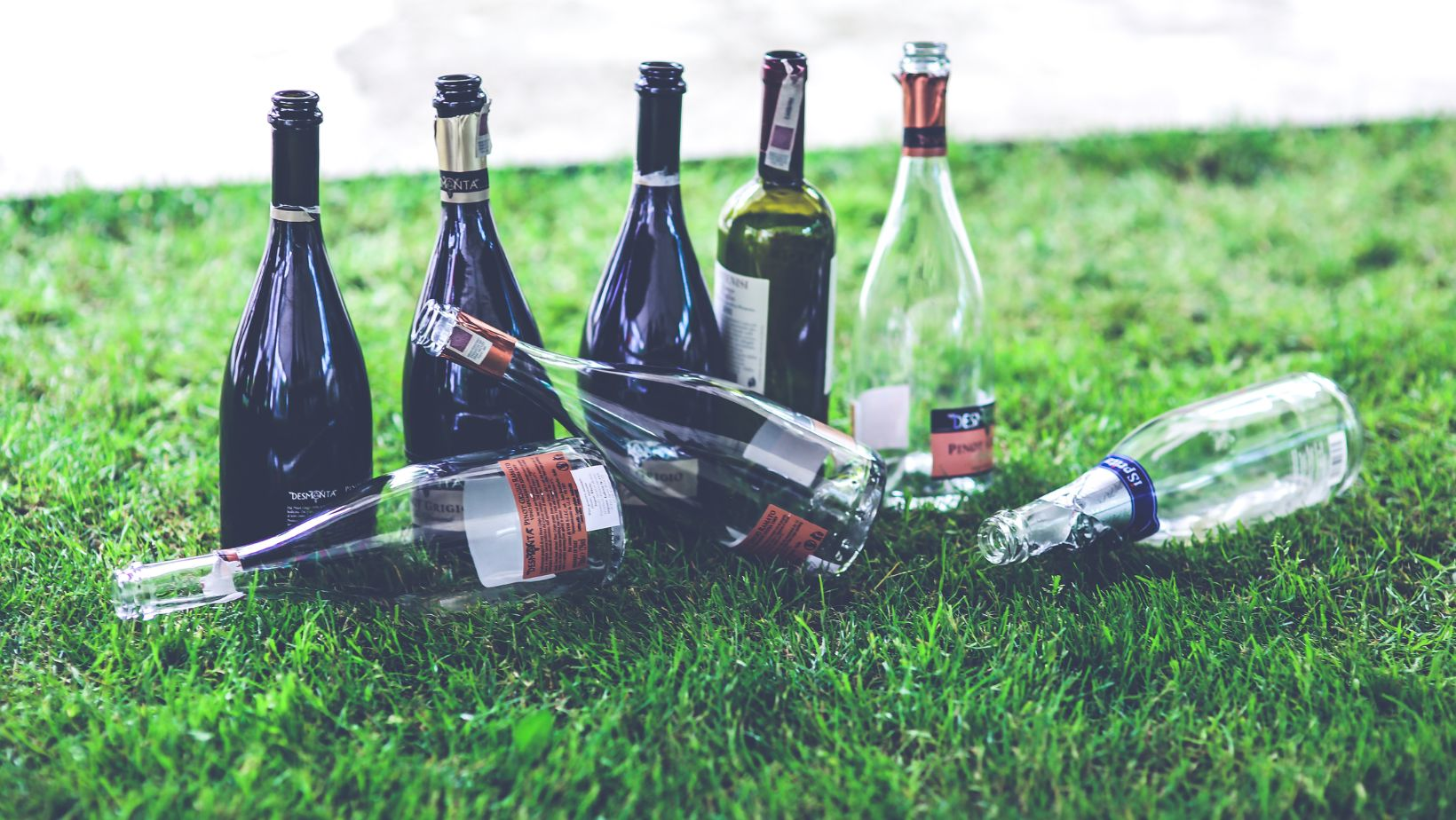# The Quick Calculation on How Many Ounces are in a 750 mL Bottle## How Many Ounces are in a 750 mL

Are you wondering about the conversion between ounces and milliliters? Specifically, how many ounces are in a 750 ml bottle? Well, I’ve got you covered! In this article, I’ll provide you with a clear answer to your question and help you understand the relationship between these two units of measurement.

So, let’s get straight to it. In terms of fluid ounces, there are approximately 25.36 ounces in a 750 ml bottle. This is an important conversion to know if you frequently work with both imperial (ounces) and metric (milliliters) measurements.

Understanding this conversion can be particularly useful when dealing with recipes or alcohol volumes. Whether you’re trying out a new recipe that requires precise measurements or simply want to know how much wine is left in that standard-sized bottle, knowing the exact conversion makes life a whole lot easier.

## What is the Conversion of Milliliters to Ounces?

When it comes to converting milliliters (ml) to ounces, the process may seem a bit perplexing at first. However, with a little understanding, you’ll be able to confidently convert between these two measurements. So let’s dive in and demystify this conversion!

Firstly, it’s essential to know that both milliliters and ounces are units of volume measurement. Milliliters are commonly used in the metric system, while ounces are more prevalent in the imperial system. To convert from milliliters to ounces, we need to establish a conversion factor.

The standard conversion factor for milliliters to ounces is 1 ml = 0.033814 oz. This means that there are approximately 0.033814 ounces in one milliliter. To calculate the number of ounces in a given amount of milliliters, you simply multiply the number of milliliters by this conversion factor.

For example:

• If I have 500 ml of water and want to know how many ounces that is: 500 ml * 0.033814 oz/ml ≈ 16.907 oz

It’s worth noting that when dealing with larger quantities or fractions of an ounce, decimal points may become necessary for precise conversions.

Now let’s address your specific query regarding how many ounces are in a 750 ml bottle? Using our trusty conversion factor:

• 750 ml * 0.033814 oz/ml ≈ 25.3605 oz

Therefore, a standard-sized bottle containing 750 ml holds approximately 25.36 fluid ounces.

Keep in mind that these conversions apply specifically to fluid measurements and might not accurately represent weight measurements due to differences in density between substances.

## Exploring the Relationship Between Milliliters and Ounces

In this section, we’ll delve into the fascinating relationship between milliliters (ml) and ounces (oz). It’s important to understand this connection, especially when it comes to measuring liquids or converting between different units of volume. So let’s dive right in!

1. ### Understanding Milliliters and Ounces:

Milliliters are a metric unit of volume commonly used in many parts of the world, including Europe and Australia. On the other hand, ounces are primarily used as a unit of volume measurement in the United States.

• 1 milliliter is equivalent to approximately 0.034 fluid ounces.
• Conversely, 1 fluid ounce is roughly equal to 29.57 milliliters.
1. ### Converting 750 ml to Ounces:

Now that we have a basic understanding of milliliters and ounces, let’s tackle the specific question at hand: “How many ounces are in a 750 ml?”

To convert milliliters to ounces, we can use the conversion factor mentioned earlier: 1 fluid ounce equals approximately 29.57 milliliters.

By applying this conversion factor to our given value of 750 ml, we can calculate:

| Milliliters | Fluid Ounces |

|————-|————–|

|    750 ml   |   X fl oz    |

To find X (the equivalent value in fluid ounces), we divide 750 by the conversion factor:

X = (750 ml) / (29.57 ml/fl oz)

Calculating this expression gives us an approximate value for X:

X ≈ 25.36 fl oz

Therefore, there are approximately 25.36 fluid ounces in a quantity of 750 milliliters.

1. ### Practical Applications:

Understanding how different units relate to each other can be incredibly useful in various scenarios, such as:

• Cooking and baking: Following recipes that use different unit measurements.
• International travel: Navigating grocery stores or understanding beverage sizes in foreign countries.
• Scientific experiments: Accurate measurement conversions for precise calculations.

By familiarizing ourselves with the relationship between milliliters and ounces, we can confidently navigate these situations and ensure accurate measurements every time.

Paige is a loving wife and an excellent chef. She owns Justalittlebite.com, a website that shares recipes and cooking tips. Paige loves spending time in the kitchen, where she can experiment with new flavors and techniques. Her husband appreciates her delicious cooking, as do her many friends and followers online. When she's not in the kitchen, Paige enjoys spending time with her family and friends.